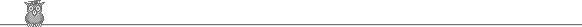### 34th Putnam 1973Problem B1

S is a finite collection of integers, not necessarily distinct. If any element of S is removed, then the remaining integers can be divided into two collections with the same size and the same sum. Show that all elements of S are equal.

Solution

Note that we need the collections to be both the same size and the same sum. Otherwise we could take S = {1, 1, 1, 1, 3}. If we remove a 1 we have: 3 = 1 + 1 + 1, and if we remove the 3 we have: 1 + 1 = 1 + 1.

The key is to notice that if we transform each element x of S to ax + b, then the new elements still satisfy the property given. In particular, by taking b sufficiently large we may make each element of S positive. Let f(S) denote the sum of the elements of S. Let us say that a finite set has the property X if (1) all its elements are positive and integral, (2) it has the property given in the question, and (3) not all its elements are equal. So we have just established that if there is a set with the property given and not all elements equal, then there is a set with property X. We show that given such a set S we can find another such set T with f(T) < f(S).

For each element x of S, f(S) - x is even, so all elements of S have the same parity. If they are all even, we may take T to be {x/2 for x in S}. If they are all odd, we may take T to be { (x + 1)/2 for x in S}. Either way, the transformed elements are all integral and positive and have the property given. Also unequal elements transform into unequal elements. So T has the property X. We always have x/2 < x, and we have (x+1)/2 < x unless x = 1, in which case (x+1)/2 = x. The elements cannot all be 1 (because we are assuming they are not all equal). So at least one must be reduced by the transformation, and hence the f(T) < f(S).

But this process could now be repeated indefinitely. That gives a contradiction, since positive integers cannot be indefinitely reduced and remain positive. Hence our assumption that all members were unequal must be wrong.

Comment. A much more interesting question is to prove the same result where we allow the elements to be reals. However, even as stated this question was curiously hard for a B1 (normally almost trivial). Or maybe I am missing a much more obvious solution - maybe good for the reals also?